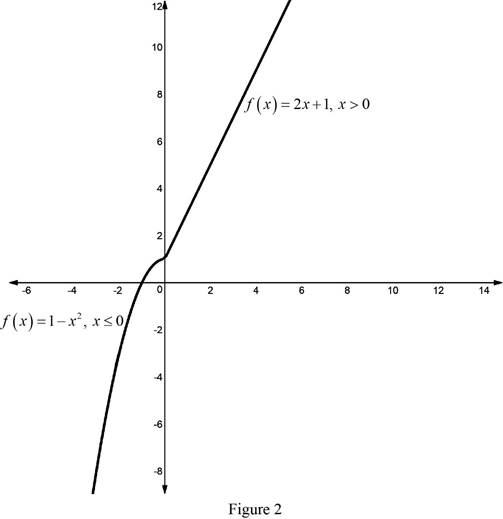# The value of f ( − 2 ) and f ( 1 ) .### Single Variable Calculus: Concepts...

4th Edition
James Stewart
Publisher: Cengage Learning
ISBN: 9781337687805### Single Variable Calculus: Concepts...

4th Edition
James Stewart
Publisher: Cengage Learning
ISBN: 9781337687805

#### Solutions

Chapter T, Problem 6CDT

a)

To determine

## To evaluate: The value of f(−2) and f(1).

Expert Solution

The values of f(2) is 3 and f(1) is 3.

### Explanation of Solution

Given;

The functions is f(x)={1x2ifx02x+1ifx>0.

Calculation:

Consider the given functions f(x)={1x2ifx02x+1ifx>0.

The value of x=2 is less then zero. So, the function is f(x)=1x2.

Substitute x=2 in f(x)=1x2,

f(2)=1(2)2=14=3

Thus, the value of f(2) is 3.

The value of x=1 is greater then zero. So, the function is f(x)=2x+1.

Substitute x=1 in f(x)=2x+1,

f(1)=2×1+1=2+1=3

Thus, the value of f(1) is 3.

b)

To determine

### To sketch: The graph of f

Expert Solution

The value of gf is 2x2+4x5.

### Explanation of Solution

Given;

The functions is f(x)={1x2ifx02x+1ifx>0.

Calculation:

The graph for the given function f(x)={1x22x+1 is shown below in Figure 1.From figure 1 it is observed that, the graph of the function f(x) has an enclosed region on the negative x-axis.

The graph for the given function f(x)={1x2ifx02x+1ifx>0 is shown below in Figure 2.From Figure 2, it is observed that the graph of the function f(x)={1x2ifx02x+1ifx>0 is a continuous curve.

### Have a homework question?

Subscribe to bartleby learn! Ask subject matter experts 30 homework questions each month. Plus, you’ll have access to millions of step-by-step textbook answers!